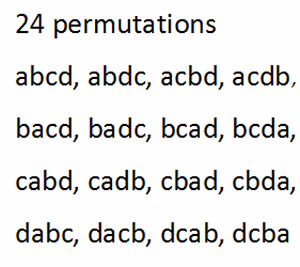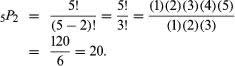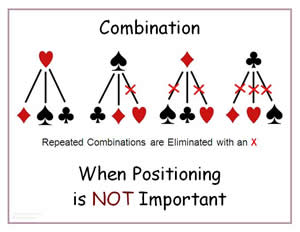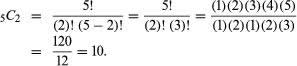# Difference between Permutation and Combination

##### Key difference: Permutation and Combination are mathematical concepts. They are different ways in which the objects may be selected from a set to form subsets. This selection of subsets is called a permutation when the order of selection is a factor, and a combination when the order is not a factor.

Permutations and combinations are both related concepts. As mathematical concepts, they serve as precise terms and language to the situation they are describing. Though they have a similar origin, they have their own significance. In general, both are related to the ‘arrangements of objects’. However, a slight difference makes each constraint applicable in different situations. This article differentiates between the two mathematical terms.A permutation is an arrangement of objects, without repetition and in which the order of the objects are important. Another definition of permutation is the total number of different arrangements that are possible by using the objects. The mathematical formula is given as:

P(n,r) = n! / (n-r)!

Since, a permutation is the number of ways one can arrange the objects, it is always a whole number. The denominator in the formula always divides evenly into the numerator. The value of ‘n’ is the total number of objects to choose from. The value of ‘r’ is the total number of given objects in the problem.

The expression n!, read “n factorial”, indicates that all the consecutive positive integers from 1 upto and including the ‘n’ object are to be multiplied together, and ‘0!’ is defined to equal 1. For example, using this formula, the number of permutations of five objects taken two at a time is(For k = n, nPk = n! Thus, for 5 objects there are 5! = 120 arrangements.)A combination is an arrangement of objects, without repetition, and in which the order of the objects is not important. Another definition of combination is the total possible number of different combinations or arrangements of all the given objects. The mathematical formula is given as:

C(n,r) = n! / ((n-r)! r!)

The ‘n’ and ‘r’ in the formula stand for the total number of objects to choose from and the number of objects in the arrangement, respectively.

In the above formula, the number of such subsets is denoted by nCr, read “n choose r.” here, since r objects have r! arrangements, there are r! indistinguishable permutations for each choice of r objects; hence there is dividing of the permutation formula by r! This formula is similar to the binomial theorem. The number of combinations of five objects taken two at a time is taken as,Comparison between Permutation and Combination:

 Permutation Combination Definition It is the selection of objects, values, and symbols with careful attention to the order, sequence, or arrangement. It is the selection of objects, symbols, or values from a large group or a certain set with underlying similarities. Importance The importance is given to the objects’ specific placement in respect to each other. The importance is on the choice of the objects or values themselves. Order The values are in order or arranged. The values are not in order or specific arrangement. Reference It is often regarded as ordered elements. They are referred to as sets. Number A number of permutations can be derived from a single combination. One combination can be derived from a single arrangement. Comparison A single permutation is distinct and different on its own and from each arrangement. A combination is often alike when compared with other combinations.